## Figures index

#### Aziz Chouikh, Rania Yousfi, Chehir Chehibi

Journal of Finance and Economics. 2017, 5(6), 300-309 doi:10.12691/jfe-5-6-6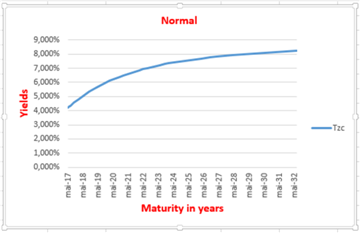• Figure 1. Normal Yield curve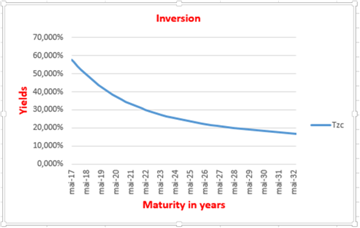• Figure 2. Inversion Yield curve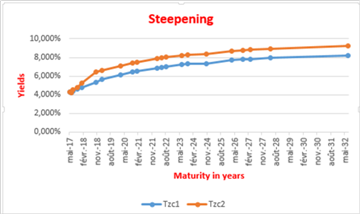• Figure 3. Steepening yield curve• Figure 4. Flatting Yield Curve• Figure 5. risk-based yield curve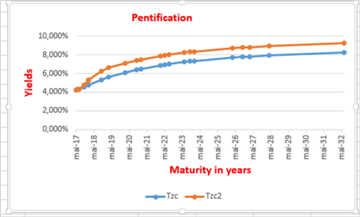• Figure 6. The steepening of the yield curve• Figure 7. Inversion yield curve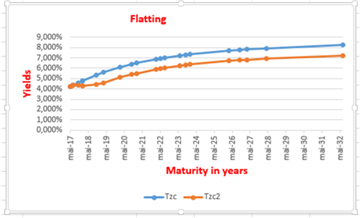• Figure 8. Flatting yield curve• Figure 9. Nelson-Siegel yield curve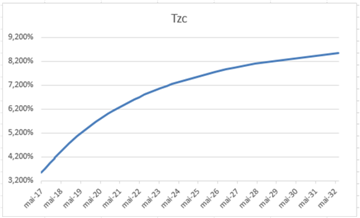• Figure 10. Svensson yield curve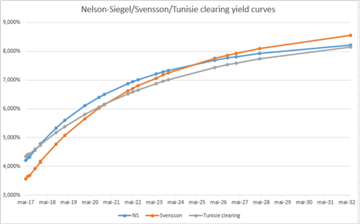• Figure 11. Nelson-Siegel, Svensson and Tunisie clearing yield curves